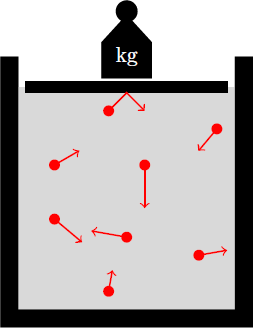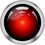# Atmospheric physics 4: Ideal gasDry air behaves in good approximation as an ideal gas and therefore follows the general gas equation: $\boxed{P = R \dfrac{n}{V} T = R^\ast \rho T}$ where

• $P$ is the pressure
• $n$ is the amount of substance (in units of mols)
• $V$ is the volume
• $\rho = M n/V$ is the mass density with the molar mass $M$
• $T$ is the absolute temperature (in units of Kelvin)
• $R \approx 8.314 \,\text{J}/\text{mol}\,\text{K}$ is general gas constant
• $R^\ast = R/M$ is the specific gas constant

Ideal gases have a low particle density, so there is virtually no interaction between the molecules (except for rare collisions). Thus, the gas particles have random positions and velocities, that are completely uncorrelated. Therefore, the thermodynamic properties of the ideal gas can be derived from the kinetic theory of gases. Here we briefly outline the arguments that can be used to derive the general gas equation. The temperature $T \propto \langle E_\text{kin} \rangle$ of the gas is a measure of the kinetic energy of the individual particles. The pressure on the walls of the container arises because the molecules regularly hit the walls, each transmitting an impulse per second, which is proportional to its kinetic energy. The pressure generated by this collisions is thus proportional to the temperature and the (mass) density of the gas. Thus, the general gas equation results except for a constant pre-factor:

$\text{pressure } P = (\text{constant}) \cdot (\text{density } \rho) \cdot (\text{temperature } T)$Note by Markus Michelmann
3 years, 3 months ago

This discussion board is a place to discuss our Daily Challenges and the math and science related to those challenges. Explanations are more than just a solution — they should explain the steps and thinking strategies that you used to obtain the solution. Comments should further the discussion of math and science.

When posting on Brilliant:

• Use the emojis to react to an explanation, whether you're congratulating a job well done , or just really confused .
• Ask specific questions about the challenge or the steps in somebody's explanation. Well-posed questions can add a lot to the discussion, but posting "I don't understand!" doesn't help anyone.
• Try to contribute something new to the discussion, whether it is an extension, generalization or other idea related to the challenge.
• Stay on topic — we're all here to learn more about math and science, not to hear about your favorite get-rich-quick scheme or current world events.

MarkdownAppears as
*italics* or _italics_ italics
**bold** or __bold__ bold
- bulleted- list
• bulleted
• list
1. numbered2. list
1. numbered
2. list
Note: you must add a full line of space before and after lists for them to show up correctly
paragraph 1paragraph 2

paragraph 1

paragraph 2

[example link](https://brilliant.org)example link
> This is a quote
This is a quote
    # I indented these lines
# 4 spaces, and now they show
# up as a code block.

print "hello world"
# I indented these lines
# 4 spaces, and now they show
# up as a code block.

print "hello world"
MathAppears as
Remember to wrap math in $$ ... $$ or $ ... $ to ensure proper formatting.
2 \times 3 $2 \times 3$
2^{34} $2^{34}$
a_{i-1} $a_{i-1}$
\frac{2}{3} $\frac{2}{3}$
\sqrt{2} $\sqrt{2}$
\sum_{i=1}^3 $\sum_{i=1}^3$
\sin \theta $\sin \theta$
\boxed{123} $\boxed{123}$

## Comments

There are no comments in this discussion.

×

Problem Loading...

Note Loading...

Set Loading...